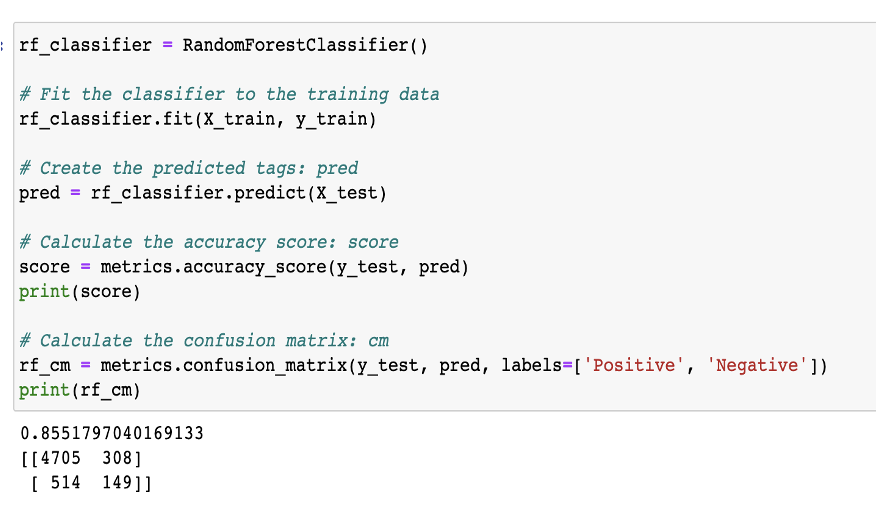top of page
Search

# Women’s E-Commerce Review Dataset (Data Analysis)

## Women’s E-Commerce Review Dataset

Includes 23486 rows and 10 unique features

Clothing ID: refers to the specific piece of clothing being reviewed

Age: refers to the reviewer’s age

Title: string variable for the title of the review

Review Text: string variable which includes the text of the review

Rating: score granted by the customer 1 being Worst and 5 being Best review

Recommended IND: binary variable describing if the customer recommends the product or not. 1 = recommend / 0 = not recommended

Positive Feedback Count: documents the number of customers who found the review positive

Division Name: categorical variable describing the division of the product

Department Name: categorical variable describing the department of the product

Class Name: Categorical variable describing the class of the product

## Purpose of the Analysis

Goal: To perform Sentiment Analysis on the women’s clothing dataset in order to classify if a review is positive or negative

Allows the customer to better understand the product

## Data Wrangling

Deleted ‘ID’ variable from the dataset

Identified and eliminated all rows with missing data

Due to the large number of categorical data eliminating the rows with missing values was more beneficial than replacing the missing values with the most common category per each variable.

## Data Visualization• Pie Chart of Rating count

• Over half of the reviews were given a 5-star rating

• Over 75% of the reviews received at least a 4-star review• Count plot of reviews by age

• Most reviews were written by customers in their 40’s

• Reviews decreased as age of the reviewer decreased after 40• Count Plot of Recommended IND

• Recommended = 82%

• Not Recommended = 18%

• Assumption: 75% of reviews received 4 or 5 star ratings and 82% of the reviews recommended the product therefore we can assume the recommended Ind variable correlates with the rating review.• Reviews per Department

• The tops department received the most reviews• Reviews per Class

• The dress class received the most reviews• Count plot of ratings per department and rating per class

• Each department and class follow the same trend with most of the reviews per department/class are 5-star reviews and the least being 1-star reviews

• Since the trend is the same per each department/class using this variable in the predictive model may not be as impactful• Eliminated 3-star reviews from the data set

• 3-star ratings are considered neutral and are not beneficial predicting positive/negative reviews

• Changed all reviews above 3 stars to positive reviews and reviews below 3 stars as negative reviews

• After eliminating the 3-star reviews 88% of the reviews were classified as positive reviewsFor each classifier the accuracy, precision and recall were calculated to determine which model had the best performance

Accuracy = (TN + TP)/(TN+TP+FN+FP)

The portion of the correctly predicted sentiment to the total number of predicted sentiment

Precision = TP/(TP+FP)

The proportion of positive sentiment were identified correctly

Recall = TP/(TP+FN)

The proportion of actual positives were correctly identified### Naïve Bayes Classifier

This classifier is based on the Bayes Theorem with the assumption of independence between every pair of features.

Results

• Accuracy = 88%

• Precision = 100%

• Recall = 88.3%### K-Nearest Neighbor Classifier

This classifier is computed by a simple majority vote of the K-nearest neighbor of each point

Results

• Accuracy = 87%

• Precision = 97%

• Recall = 90%### SGD Classifier

SGD classifier is an efficient approach to fit linear models. More useful in large datasets

Results

• Accuracy = 86%

• Precision = 91%

• Recall = 92%### Decision Tree Classifier

The decision tree classifier is a classifier that predicts the value of a target variable by learning simple decision rules inferred from the data features

Results

• Accuracy = 81%

• Precision = 88%

• Recall = 91%### Logistic Regression Classifier

This logistic regression classifier is a classification model which is expressed in the form of conditional probability distribution

Results

• Accuracy = 89%

• Precision = 99%

• Recall = 89%### Random Forest Classifier

The random forest classifier is an ensemble learning method for classification. The output of this model is the class selected by most trees

Results

• Accuracy = 85%

• Precision = 94%

• Recall = 90%### Comparison of Model Performance

Logistic Regression model received the highest accuracy of all the models

Naïve Bayes received the highest Precision score

## Solution

• The goal was to perform Sentiment Analysis on the women’s clothing dataset in order to classify customer review text using machine learning models

• 6 models were chosen to classify the customer reviews focusing on three variables which included the ‘Review Text’ , ‘Age’ and ‘Rating’ of the reviews

• the results show that the logistic regression classifier was the best classifier for this dataset. Not only did the logistic regression receive the highest accuracy it also received the second highest precision score

• Moving forward in order to enhance the accuracy of classifying women’s clothing reviews more variables should be considered for the dataset

• Region, Salary and occupation of a customer are a few examples of variables that could improve the accuracy of classifying customer reviews through machine learning models

Research Provided by Andrey Fateev

7 views

See All
bottom of page